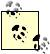# Using Trigonometry Functions

Problem

You need to become familiar with Excel's support for trigonometric functions .

Solution

Explore the trigonometric functions summarized in Table 7-5.

Table 7-5. Trigonometric functions

Function

Syntax

Description

SIN

=SIN(angle)

Returns the sine of the given angle, where angle is in radians.

COS

=COS(angle)

Returns the cosine of the given angle, where angle is in radians.

TAN

=TAN(angle)

Returns the tangent of the given angle, where angle is in radians.

ASIN

=ASIN(n)

Returns the inverse sine of the given number, n, where n must be within the range -1 to 1. The returned angle is in radians and within the range -p/2 to p/2.

ACOS

=ACOS(n)

Returns the inverse cosine of the given number, n, where n must be within the range -1 to 1. The returned angle is in radians and within the range 0 to p.

ATAN

=ATAN(n)

Returns the inverse tangent of the given number, n. The returned angle is in radians and within the range -p/2 to p/2.Excel's function PI( ) returns the value of p (i.e., 3.14159265358979...), which you might find useful in some calculations.

Discussion

The trigonometric functions take or return angles in radians. However, often degrees are more convenient to work with. Excel provides two functions making conversions between radians and degrees easy: RADIANS and DEGREES. Use RADIANS(angle) to convert an angle from degrees to radians. Use DEGREES(angle) to convert an angle from radians to degrees. For example, =COS(RADIANS(45)) returns the cosine of the angle 45 degrees.Excel Scientific and Engineering Cookbook (Cookbooks (OReilly))
ISBN: 0596008791
EAN: 2147483647
Year: N/A
Pages: 206
Authors: David M Bourg